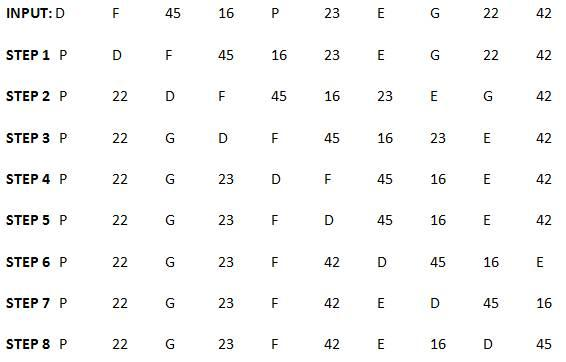Top

# Sequential Output Tracing

##### Direction for [ Question No: 1 To 5 ] :

Study the following information carefully to answer the given questions.

A word and number arrangement machine when given an input line of words and numbers rearranges them following a particular rule in each step. The following is an illustration of an input and its rearrangement.

Input:done with 41 17 one 13 front 24
Step I:with done 41 17 one 13 front 24
Step II: with 13 done 41 17 one front 24
Step III: with 13 one done 41 17 front 24
Step IV: with 13 one 41 done 17 front 24
Step V: with 13 one 41 front done 17 24
Step VI: with 13 one 41 front 24 done 17

And step VI is the last step of the above input. As per the rules followed in the above step, find out the appropriate step for the above input.

Input: daughter female 45 16 painter 23 elder grand 22 42

1.

The following output will be in which step?
Painter 22 grand 23 female daughter 45 16 elder 42

STEP V
Words – are descending alphabetical order
Numbers – sum of the two digits of each numbers are arranged in ascending order#### Enter details here

2.

How many steps will be required to complete the given rearrangement?

Eight steps Required to complete
Words – are descending alphabetical order
Numbers – sum of the two digits of each numbers are arranged in ascending order.#### Enter details here

3.

Which of the following represents the first two and the last two elements in the last step?

Painter, 22 and Daughter 45

Words – are descending alphabetical order

Numbers – sum of the two digits of each numbers are arranged in ascending order.#### Enter details here

4.

Which of the following elements is fifth from the left in step V?

Fifth element from left in Step 5 is Female

Words – are descending alphabetical order

Numbers – sum of the two digits of each numbers are arranged in ascending order.#### Enter details here

5.

How many elements are there between 42 and 45 in step VII?